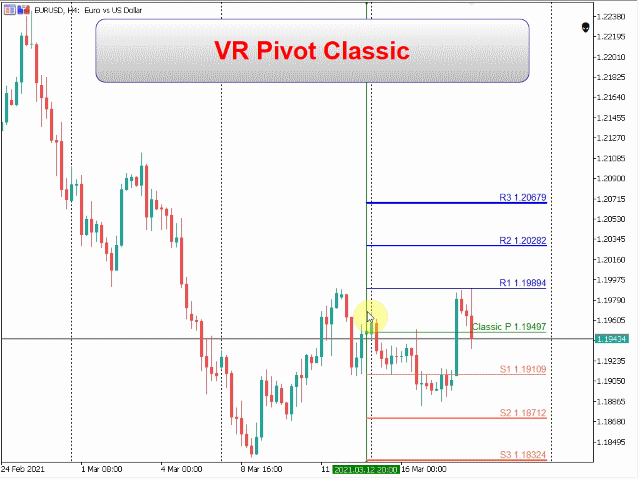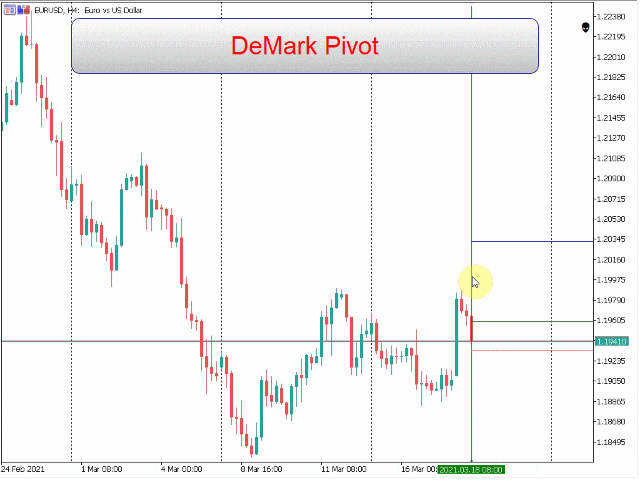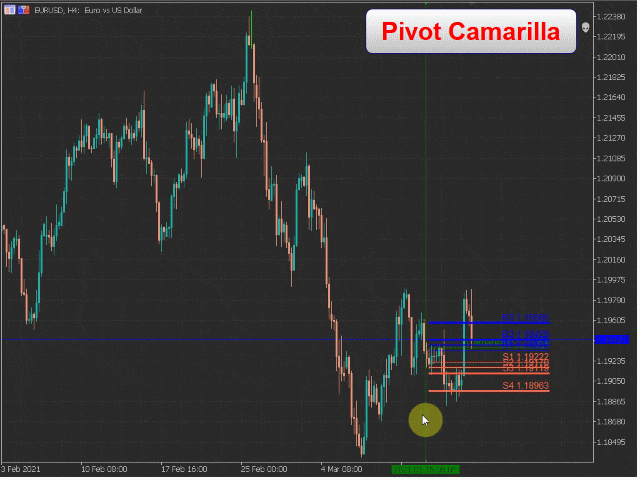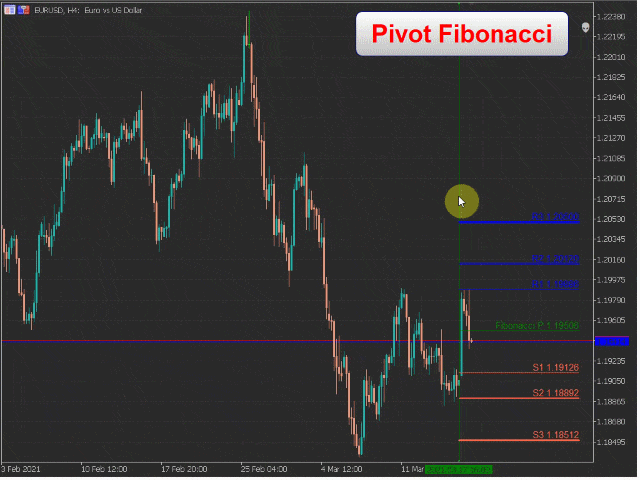# VR Pivot MT5VR Pivot MT5

⚡ VR Pivot is an indicator of key pivot points.

Pivot points are a special type of support and resistance levels that are used to determine the strongest price zones. Professional traders use pivot levels to determine prices at which there is a high probability of a price reversal. The levels are calculated using special mathematical formulas, which include the closing, opening, high, and low prices of the previous time period. The most effective is the daily period. It is important to note that at first glance it may seem that there is nothing special about these levels, but if you analyze the price movement using pivot levels, you can clearly see that in most cases these levels show good, accurate results.

Pivot levels can be used not only as pivot points, but also as levels at which Take Profit can be used as efficiently as possible to maximize profit, Stop Loss to minimize losses as much as possible, and transfer positions to a no-loss zone to reduce risks.

Advantages of the VR Pivot Indicator:
The indicator supports the calculation of several formulas:
Classic Pivot – the classic formula for pivot points
DeMark Pivot – DeMark formula
Woodie Pivot – Woodie Formula
Camarilla Pivot – Camarilla Formula
Fibonacci Pivot – Fibonacci Formula
The program is implemented as an indicator and can work together with your Expert Advisors and other indicators
It is possible to analyze historical data
The program is written in such a way that it displays information for the period set by the user and does not clutter the chart with historical data.
The indicator can calculate losses for any financial instruments (Forex, Crypto, CFD, Futures, Metals)
The trader can choose any time frames for the calculation
The indicator has a large number of settings that allows you to customize it in any style
Indicator operation algorithm
After you drag the indicator from the navigator to the chart, you will see a settings window where you can select the calculation formula and adjust the colors of the levels. After you click the “Ok” button, the chart will display horizontal levels and one vertical level. Using the vertical level, the trader can move the starting point of the calculations. Use the vertical level to study the historical data. To calculate new key levels, move the vertical level to the current period.

📌 Indicator Settings
Main settings – main indicator settings
Type Pivot – type of pivots
Classic Pivot – the classic formula for pivot points
DeMark Pivot – DeMark formula
Woodie Pivot – Woodie Formula
Camarilla Pivot – Camarilla Formula
Fibonacci Pivot – Fibonacci Formula
Length of lines – the length of horizontal levels in bars
Show Price – display prices on levels
Time Frame – The period for calculating the levels, must be greater than the period for which the indicator is set
Prefix – prefix for indicator objects
Color settings – indicator color settings
Full version VR Pivot
Demo version VR Pivot Demo
Full version VR Pivot
Demo version VR Pivot Demo